# Calculate the median by using Transact SQL

Total: 9 Average: 4.1

The statistical median is the value which separates a dataset into halves – one comprises greater values, and the other comprises lesser ones. For a specified dataset, it can be considered as the “middle” value. For example, in the dataset {1, 3, 3, 4, 5, 6, 7, 8, 9}, the median is 5, which is fourth largest, and fourth smallest number in the dataset.

To calculate the median of any dataset, we first need to arrange all values from the dataset in a specific order. After arranging the data, we must determine the middle value of the specified dataset. If the dataset contains an odd number of values, than the middle value of the entire dataset will be considered as a median. Following is the example:

Median (M) = value of ((X + 1)/2) th item. (x is the number of values in the dataset)

For example, if we calculate the median of the dataset {1,2,3,4,6,7,8} by using the above formula, then Median (M)= (7+1)/2=4. So, the fourth value of the specified dataset, which is 4 in this case, is the median.

If the dataset contains an even number of rows, we must determine the two middle values of this dataset, add them together, and divide the result by two. The following formula is used to calculate the median:

Median (M) = value of [(x/2) th item + (x/2 + 1) th item]/2 (x is the number of values in the dataset)

For example, if we apply this formula to the dataset {1,2,4,6,8,10}, then the median value is calculated as shown below:

Median (M)= [ 6/2 ] = 3rd value of the dataset + [ 6/2 + 1 ]= 4th value of the dataset.

= (4+6)/2 = 5. So, the median value in this case is 5.

Usually, it is difficult to calculate the median value of a specified dataset by using Transact SQL because there is no built-in function available for this purpose. But we can make it easier by using the Rank function and common table expressions.

1. Preparing the Demo setup.
2. Calculating the median by using the RANK() function and CTE (common table expressions).
3. Calculating the median by using PERCENTILE_COUNT(). It applies to SQL Server 2012 and later versions.

## Preparing the Demo setup

To demonstrate how the median can be calculated with the help of Transact SQL, let’s first prepare a demo setup. To do that, we’ll need to create a SQL Table named “Invoice.” The Invoice table contains the invoice number, Sale quantity, and the Price column. Execute the following code to create the Invoice table.

```CREATE TABLE INVOICE
(
ID            INT IDENTITY(1, 1),
INVOICENUMBER VARCHAR(50),
PRICE         NUMERIC(10, 2),
QUANTITY      NUMERIC(10, 2)
)```

Now insert some dummy data by executing the following query.

```INSERT [DBO].[INVOICE] ([ID], [INVOICENUMBER], [PRICE], [QUANTITY]) VALUES (1, N'DES1112015794', CAST(9.00 AS NUMERIC(10, 2)), CAST(1.00 AS NUMERIC(10, 2)))

INSERT [DBO].[INVOICE] ([ID], [INVOICENUMBER], [PRICE], [QUANTITY]) VALUES (2, N'DES1112015794', CAST(10.00 AS NUMERIC(10, 2)), CAST(1.00 AS NUMERIC(10, 2)))

INSERT [DBO].[INVOICE] ([ID], [INVOICENUMBER], [PRICE], [QUANTITY]) VALUES (3, N'DES1112015794', CAST(11.00 AS NUMERIC(10, 2)), CAST(1.00 AS NUMERIC(10, 2)))

INSERT [DBO].[INVOICE] ([ID], [INVOICENUMBER], [PRICE], [QUANTITY]) VALUES (4, N'DES1112015794', CAST(12.00 AS NUMERIC(10, 2)), CAST(1.00 AS NUMERIC(10, 2)))

INSERT [DBO].[INVOICE] ([ID], [INVOICENUMBER], [PRICE], [QUANTITY]) VALUES (5, N'DES1112015794', CAST(12.00 AS NUMERIC(10, 2)), CAST(2.00 AS NUMERIC(10, 2)))

INSERT [DBO].[INVOICE] ([ID], [INVOICENUMBER], [PRICE], [QUANTITY]) VALUES (6, N'DES1112015794', CAST(18.00 AS NUMERIC(10, 2)), CAST(1.00 AS NUMERIC(10, 2)))

INSERT [DBO].[INVOICE] ([ID], [INVOICENUMBER], [PRICE], [QUANTITY]) VALUES (7, N'DES1112018052', CAST(3.00 AS NUMERIC(10, 2)), CAST(2.00 AS NUMERIC(10, 2)))

INSERT [DBO].[INVOICE] ([ID], [INVOICENUMBER], [PRICE], [QUANTITY]) VALUES (8, N'DES1112018052', CAST(5.00 AS NUMERIC(10, 2)), CAST(1.00 AS NUMERIC(10, 2)))

INSERT [DBO].[INVOICE] ([ID], [INVOICENUMBER], [PRICE], [QUANTITY]) VALUES (9, N'DES1112018052', CAST(12.00 AS NUMERIC(10, 2)), CAST(1.00 AS NUMERIC(10, 2)))

INSERT [DBO].[INVOICE] ([ID], [INVOICENUMBER], [PRICE], [QUANTITY]) VALUES (10, N'DES1112018052', CAST(18.00 AS NUMERIC(10, 2)), CAST(2.00 AS NUMERIC(10, 2)))

INSERT [DBO].[INVOICE] ([ID], [INVOICENUMBER], [PRICE], [QUANTITY]) VALUES (11, N'DES1112019188', CAST(20.00 AS NUMERIC(10, 2)), CAST(5.00 AS NUMERIC(10, 2)))

INSERT [DBO].[INVOICE] ([ID], [INVOICENUMBER], [PRICE], [QUANTITY]) VALUES (12, N'DES1112019188', CAST(23.00 AS NUMERIC(10, 2)), CAST(5.00 AS NUMERIC(10, 2)))

INSERT [DBO].[INVOICE] ([ID], [INVOICENUMBER], [PRICE], [QUANTITY]) VALUES (13, N'DES1112021986', CAST(27.00 AS NUMERIC(10, 2)), CAST(1.00 AS NUMERIC(10, 2)))

INSERT [DBO].[INVOICE] ([ID], [INVOICENUMBER], [PRICE], [QUANTITY]) VALUES (14, N'DES1112022597', CAST(9.00 AS NUMERIC(10, 2)), CAST(5.00 AS NUMERIC(10, 2)))

INSERT [DBO].[INVOICE] ([ID], [INVOICENUMBER], [PRICE], [QUANTITY]) VALUES (15, N'DES1112022597', CAST(12.00 AS NUMERIC(10, 2)), CAST(1.00 AS NUMERIC(10, 2)))

INSERT [DBO].[INVOICE] ([ID], [INVOICENUMBER], [PRICE], [QUANTITY]) VALUES (16, N'DES1112022597', CAST(36.00 AS NUMERIC(10, 2)), CAST(1.00 AS NUMERIC(10, 2)))

INSERT [DBO].[INVOICE] ([ID], [INVOICENUMBER], [PRICE], [QUANTITY]) VALUES (17, N'DES1112022597', CAST(155.00 AS NUMERIC(10, 2)), CAST(1.00 AS NUMERIC(10, 2)))
INSERT [DBO].[INVOICE] ([ID], [INVOICENUMBER], [PRICE], [QUANTITY]) VALUES (18, N'DES1112024469', CAST(95.00 AS NUMERIC(10, 2)), CAST(1.00 AS NUMERIC(10, 2)))```

Execute the following query to review the data:

```SELECT INVOICENUMBER,
PRICE,
QUANTITY
FROM   [INVOICE]```

This is the output we should get: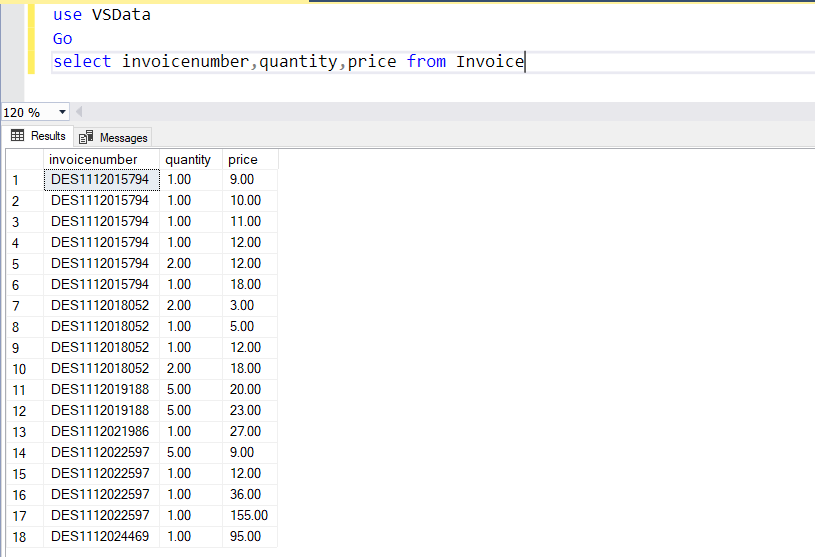Now we want to calculate the median of the price of an individual invoice number. For example, Invoice XYZ123 has more than five items, so we will generate the median price of all items in this invoice.

## Calculate the median by using the ranking function and common table expressions

If you are using SQL Server 2000/ 2005 / 2008, you can calculate the median by using 50 percent highest value and 50 percent lowest values. This method is quite simple and can be used to calculate the median value of an odd or even number of rows within a specified dataset.

To calculate this, execute the following query:

```SELECT ( (SELECT MAX(PRICE),
INVOICENUMBER
FROM   (SELECT TOP 50 PERCENT PRICE,
INVOICENUMBER
FROM   INVOICE
GROUP  BY INVOICENUMBER
ORDER  BY INVOICENUMBER) AS BOTTOMHALF)
+ (SELECT MIN(PRICE),
INVOICENUMBER
FROM   (SELECT TOP 50 PERCENT PRICE,
INVOICENUMBER
FROM   INVOICE
GROUP  BY INVOICENUMBER
ORDER  BY INVOICENUMBER DESC) AS TOPHALF) ) / 2 AS MEDIAN```

This is the output we should get: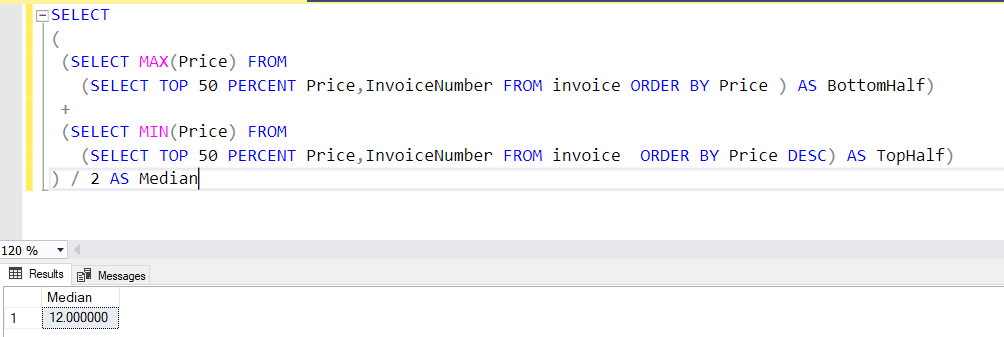The above value is the median of all rows from the invoice table. Now we want to calculate the median value of the price of items from an individual invoice number.

To do that, execute the following query:

```SELECT INVOICENUMBER,
AVG(PRICE) AS MEDIANVAL
FROM   (SELECT INVOICENUMBER,
PRICE,
ROW_NUMBER()
OVER (
PARTITION BY INVOICENUMBER
ORDER BY PRICE ASC, INVOICENUMBER ASC) AS ROWASC,
ROW_NUMBER()
OVER (
PARTITION BY INVOICENUMBER
ORDER BY PRICE DESC)                   AS ROWDESC
FROM   INVOICE SOH) X
WHERE  ROWASC IN ( ROWDESC, ROWDESC - 1, ROWDESC + 1 )
GROUP  BY INVOICENUMBER
ORDER  BY INVOICENUMBER;```

The output is as follows: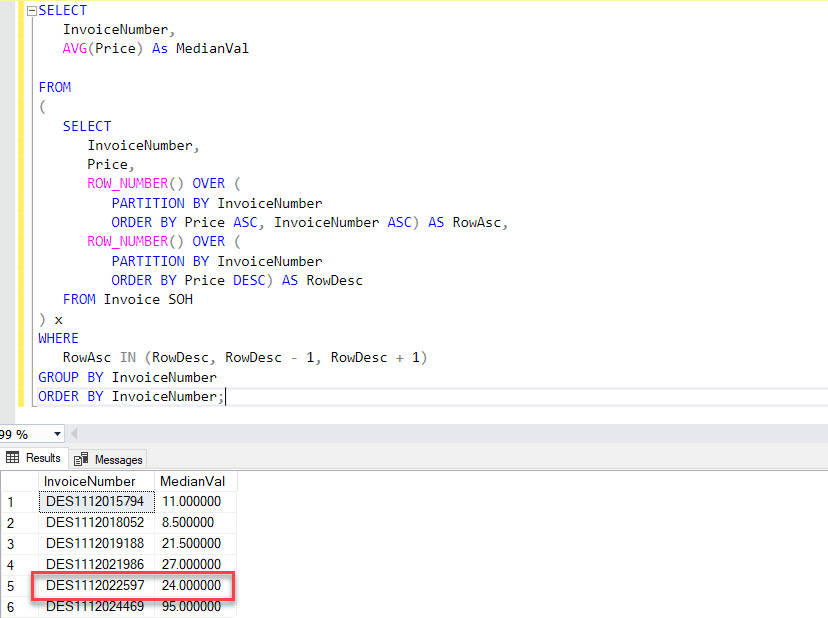As you can see in the image above, 24.00 is the median value of the Invoice “DES1112022597.”

## Calculate the median by using PERCENTILE_COUNT (SQL Server 2012 and later versions)

An analytic function called PERCENTILE_CONT was introduced in SQL Server 2012. It is capable of calculating the median within a partitioned set. It calculates the median when we pass 0.5 as an argument and specify the order within that dataset.

The following query demonstrates how to use this function:

```SELECT INVOICENUMBER,
PRICE,
PERCENTILE_CONT(0.5)
WITHIN GROUP (ORDER BY PRICE) OVER (
PARTITION BY INVOICENUMBER) AS MEDIANCONT
FROM   INVOICE
ORDER  BY INVOICENUMBER DESC```

This is the output we should get: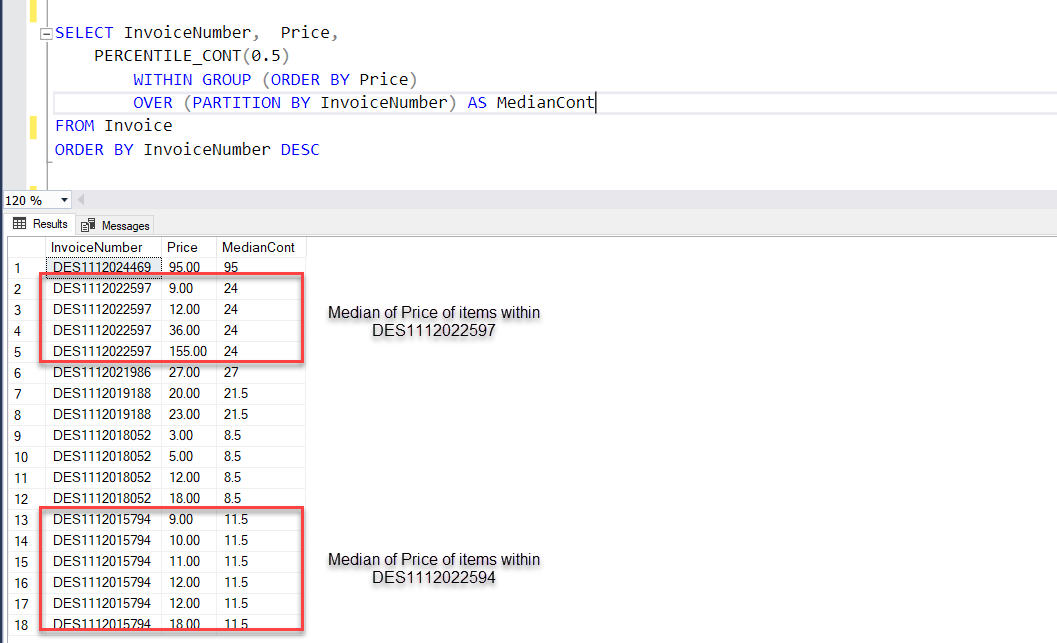As you can see in the image above, Invoice DES1112022597 has four items in total and the median value for the Price column within this invoice equals to the the sum of Price in row 3 and Price in row 4 divided by two. With the right numbers in place, we get (36+12)/2 =24.00. Similarly, the median value for invoice DES1112022594 will equal (11+12)/2 = 11.5.

## Creating a function to calculate the median value

Now, let’s create a T-SQL Function to calculate the median value of the specified dataset. This function can be used in all version of SQL Server. The procedure takes a table name and a column name as input parameters to calculate the median value for any data type. It creates a temporary table from the column values and sorts them. It uses a dynamic scrollable cursor which scrolls to the middle of the result set and gives the output.

The following is the function’s actual code:

```USE VSDATA
GO

CREATE PROCEDURE DBO.PROC_CALCULATE_MEDIAN (@TABLENAME  VARCHAR(50),
@COLUMNNAME VARCHAR(50),
@OUTPUT     SQL_VARIANT OUTPUT)
AS
BEGIN
DECLARE @SQLQUERY VARCHAR(200)
DECLARE @MIDDLE_VALUE INT
SET NOCOUNT ON
CREATE TABLE #WORKTABLE
(
COLUMNS SQL_VARIANT
)
SET @SQLQUERY = 'INSERT #WORKTABLE SELECT ' + @COLUMNNAME
+ ' FROM ' + @TABLENAME + ' ORDER BY 1 ASC '
EXEC (@SQLQUERY)
DECLARE CURSOR_MED CURSOR SCROLL FOR
SELECT *
FROM   #WORKTABLE
SELECT @MIDDLE_VALUE = ROUND (COUNT(*) * 0.5, 0)
FROM   #WORKTABLE
OPEN CURSOR_MED
FETCH ABSOLUTE @MIDDLE_VALUE FROM CURSOR_MED INTO @OUTPUT
CLOSE CURSOR_MED
DEALLOCATE CURSOR_MED
DROP TABLE #WORKTABLE
END
GO```

Let’s run this procedure and check the output. Firstly, we’ll execute this procedure to get the median value of the Prices column from the Invoice Table. To run the procedure, execute the following query:

```DECLARE @MEDIAN_VALUE SQL_VARIANT

EXEC DBO.SP_CALC_MEDIAN
'INVOICE',
'PRICE',
@MEDIAN_VALUE OUTPUT

SELECT @MEDIAN_VALUE```

This is the output we should get: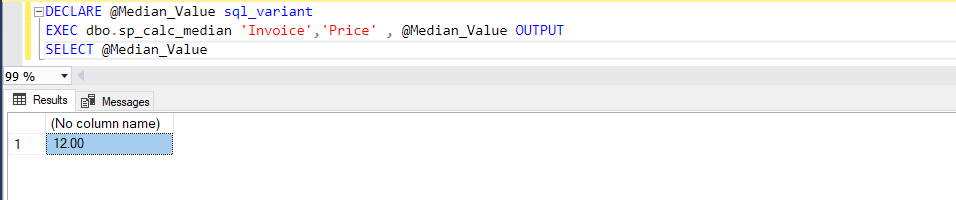We can also calculate the median of the InvoiceNumber column from the Invoices table. To do that, run the following query:

```DECLARE @MEDIAN_VALUE SQL_VARIANT

EXEC DBO.SP_CALC_MEDIAN
'INVOICE',
'INVOICENUMBER',
@MEDIAN_VALUE OUTPUT

SELECT @MEDIAN_VALUE```

We should get the following output: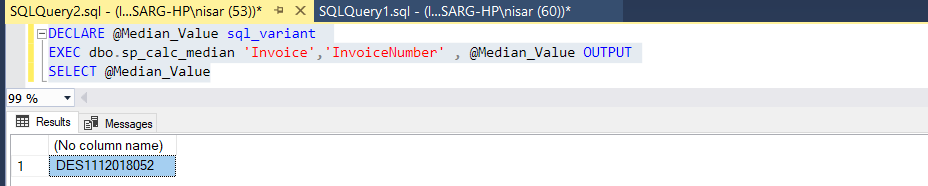## Summary